## Fourier Matrix

TheSquare Matrix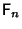with entries given by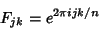(1)

for, 1, 2, ...,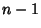, and normalized by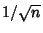to make it a Unitary. The Fourier matrixis given by(2)

and the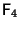matrix by(3)

In general,(4)

with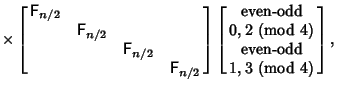(5)
whereis theIdentity Matrix. Note that the factorization (which is the basis of the Fast Fourier Transform) has two copies ofin the center factor Matrix.

Strang, G. Wavelet Transforms Versus Fourier Transforms.'' Bull. Amer. Math. Soc. 28, 288-305, 1993.